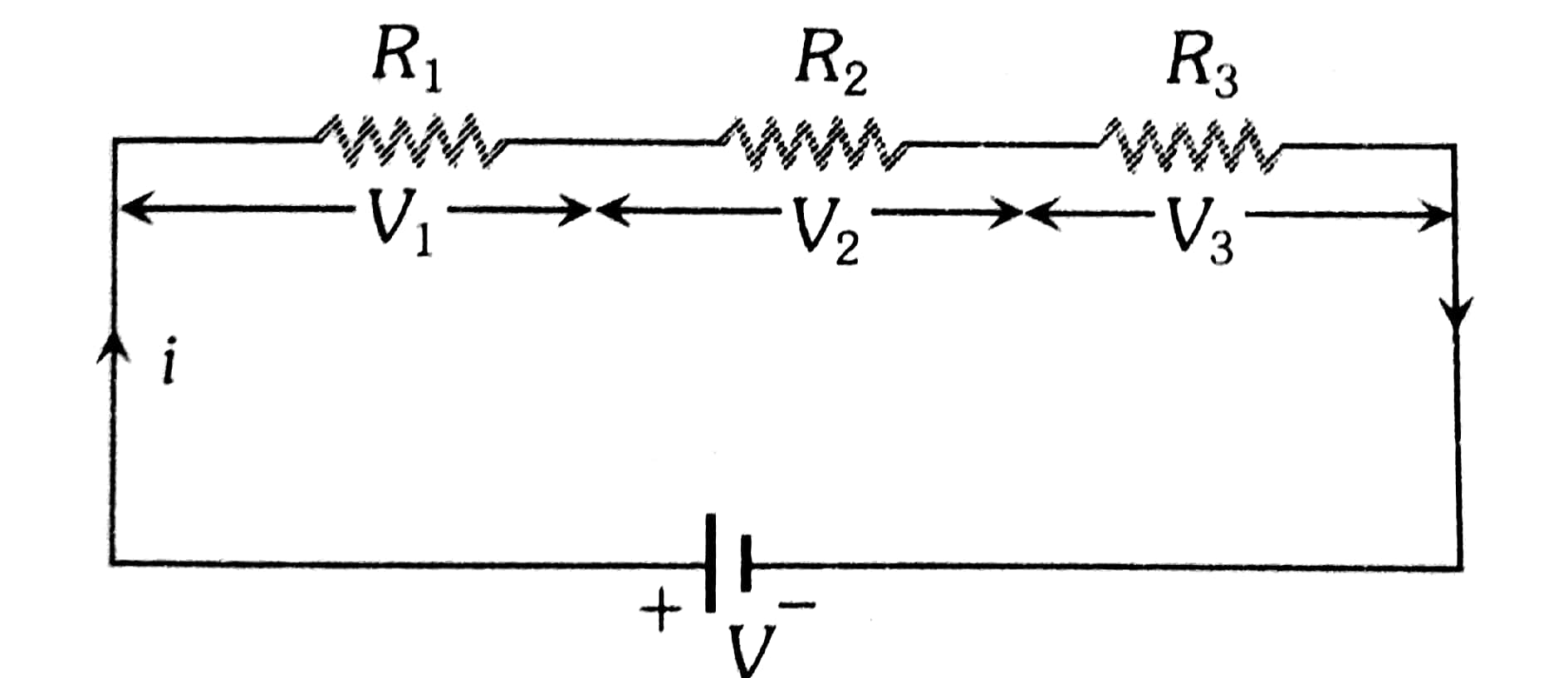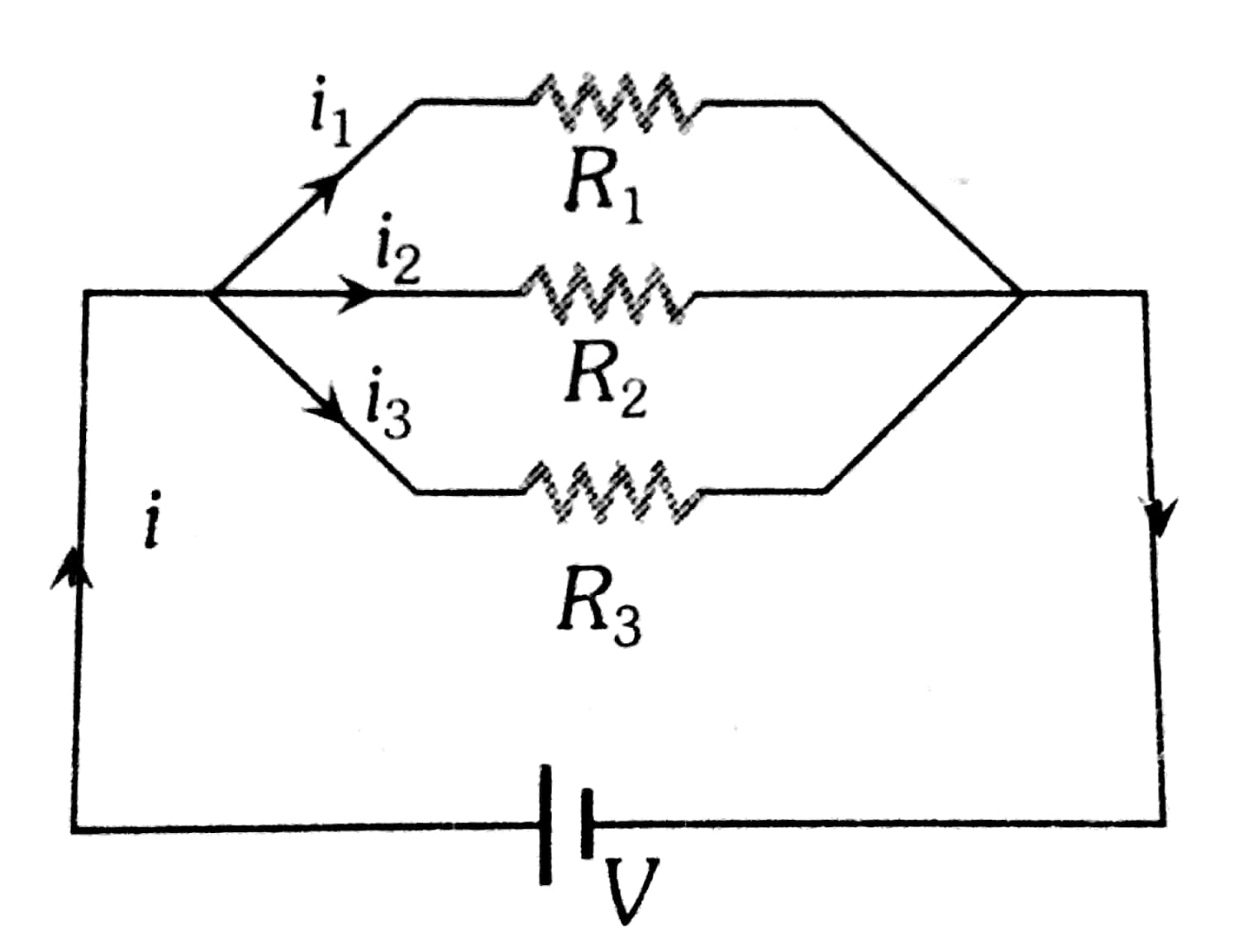Q

# Solve it, - Current Electricity - JEE Main

Two conductors have the same resistance at 0°C but their temperature coefficients of resistance are . The respective temperature coefficients of their series and parallel combinations are nearly

• Option 1)

• Option 2)

• Option 3)

• Option 4)

178 Views

As we learnt in

Temperature co-efficient of Resistivity -

- wherein

Where the value of  is different at different temperatures

Series Grouping -

Potential - Different

Current - Same

- whereinParallel Grouping -

Potential - Same

Current - Different

- whereinin series

In parallel

Option 1)

This option is correct.

Option 2)

This option is incorrect.

Option 3)

This option is incorrect.

Option 4)

This option is incorrect.

Exams
Articles
Questions